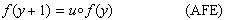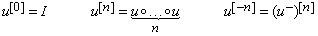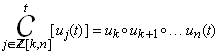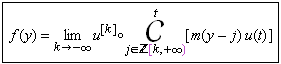# Iterative Abel Functional Equation: f(y + 1) = u∘f(y)

by Kyriakos Kefalas

There are various functional equations (FE) which were studied by Niels Henrik Abel (1802 – 1829)(1). Among them are functional equations in a single variable, which are called iterative functional equations by Marek Kuczma(2). In this article we will consider the  Abel iterative functional equation:This FE has a special significance among all functional equations, since its solutions are intricately connected with the iterates of the function u. A first long standing problem is to find continuous solutions f, for an arbitrary function u. Although the class of solutions is infinite for every particular function u, it has been difficult to find even one such solution in most cases. A second long standing problem is to characterize a unique solution among the infinite possible, through implementation of additional criteria.

In this article we will address the first of these problems. We present continuous solutions of the AFE for a wide class of functions u. For the detailed method and proofs see [Kefalas, 2014].

### Inverse and iterates of functions

For our convenience we introduce the special notation, , for the inverse of an invertible function u. Then:

where I, is the identity function. We also use  a nonstandard notation for functional iterates, to avoid confusion with powers . This is:where, .

### Continued forms

In [Kefalas, 2014] the author introduces the ‘continued form notation’, which generalizes the familiar Σ, and Π, notation. Let, , be a sequence of univariate functions and assume that the successive composition of the sequence members is possible. Then the continued form of the sequence Su,  is defined as:where t, is the variable of composition.

### Continuous solutions of the AFE

We can now give a formula for the continuous solutions of the title AFE:where, , is any invertible function such that, . As expected the solution depends on an arbitrary continuous function, . This function is called a modulator function and is defined as:

In [Kefalas, 2014] it is proved that the double limits always exist and that f, satisfies the AFE.